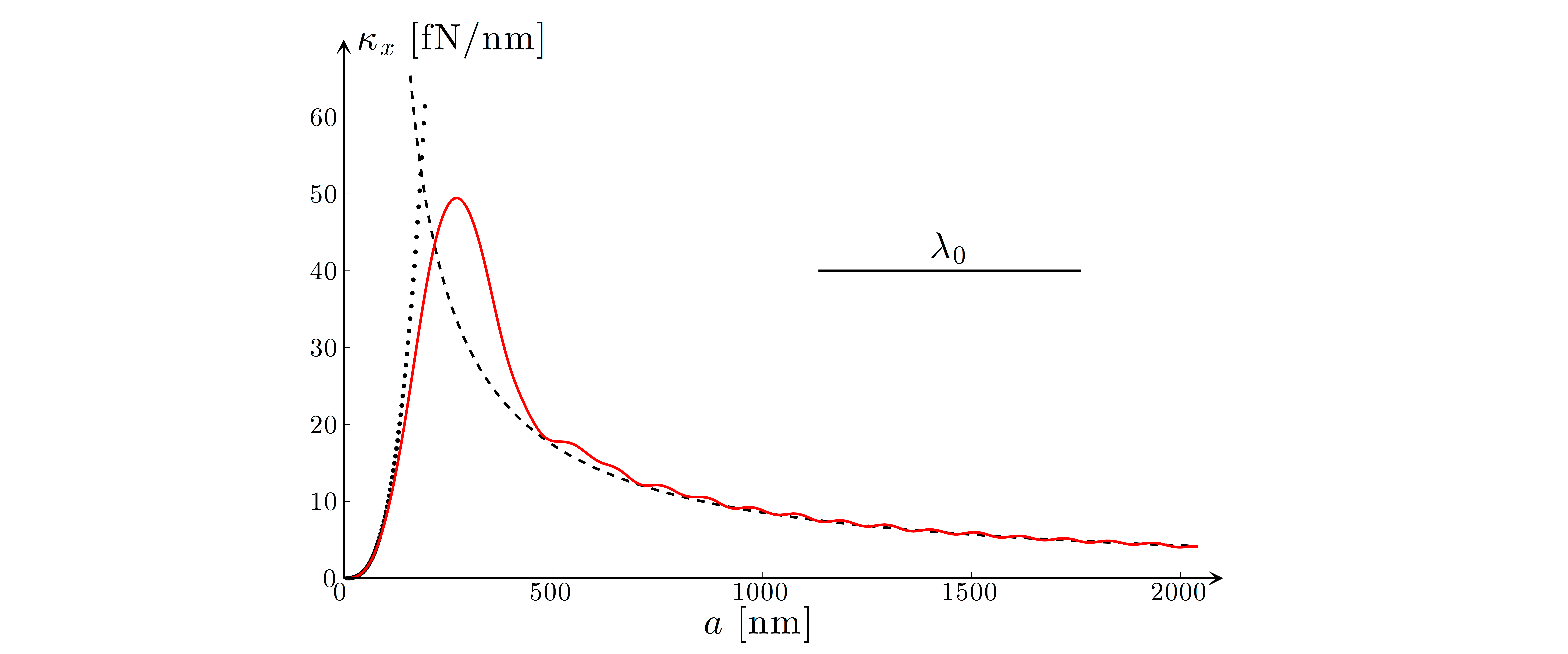# Figure 5.1 — Comparison of optical forces calculated in various trapping regimesFigure 5.1 — Comparison of optical forces calculated in various trapping regimes. Transverse trap stiffness produced by a 10 mW laser beam of wavelength λ0 = 632 nm focused by a 1.20 NA objective on a dielectric sphere of radius a (np = 1.50) in water (nm = 1.33). The solid line represents the exact electromagnetic calculation. The dipole approximation (dotted line) works for small spheres (a ≪ λ0). The geometrical optics approximation (dashed line) works for large spheres (a ≫ λ0).